You are viewing documentation for an older version. For current documentation - click here.

FusionCharts XT offers a lot of options to format numbers on the chart. You can configure number prefixes & suffixes, decimal places, and scaling of numbers based on a pre-defined scale. In this section, we will see the number formatting properties supported by FusionCharts XT and look into number scaling in the next section.

We will start with setting decimal precisions for the numbers on chart.

Configuring decimal places (rounding)

All the charts in FusionCharts XT support the attribute decimals. This single attribute lets you control the decimal precision of all the numbers on the chart. Using this attribute, you can globally set the number of decimal places of all numbers of the chart.

For example, if you have numbers on your chart as 12.432, 13.4 and 13 and you set <chart ... decimals='2' >, the numbers will be converted to 12.43, 13.4 and 13 respectively.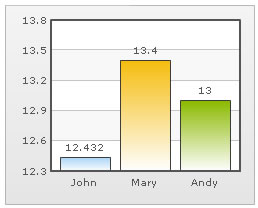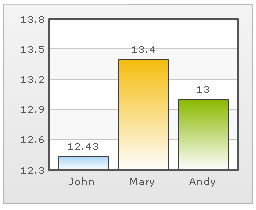Chart without any decimal formatting applied With decimals set to 2 (see the first column value)

Similarly, if you had data as 0.12352, 0.134 and 0.13, and you set decimals as 3, FusionCharts XT will output 0.124, 0.134 and 0.13 respectively. Note that even though we have set decimals to 3, FusionCharts XT now does not forcibly add the 0 padding to 0.13 to form 0.130, as the trailing 0 is un-necessary.

However, if you want your numbers on the chart to have trailing zeroes too, you can set <chart ... forceDecimals='1' > and now the numbers will show as 0.124, 0.134 and 0.130 respectively. Shown below are examples: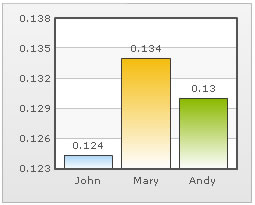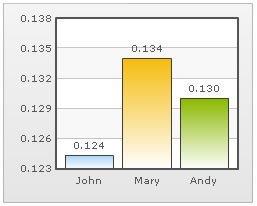Trailing zeroes missing in last column's value Forcing trailing zeroes by setting forceDecimals='1'

On Dual y-axis charts, you have to set decimals and configuration per-axis. So, the XML/JSON will look like:

`<chart decimals='3' sDecimals='2' forceDecimals='1'  >`
` "chart":{    "decimals":"3",    "sdecimals":"2",    "forcedecimals":"1"  }`

Here, sDecimals refers to the decimal places to be added to any data point plotted against secondary axis (hence the prefix s before decimals).

Automatic number formatting

FusionCharts XT automatically formats your numbers by adding K,M (Kilo, Million) and proper commas to the numbers. Shown below is an example: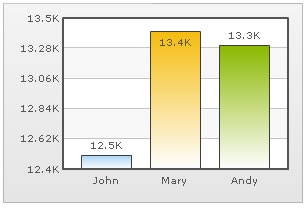In the above image, the data for chart is 12500, 13400 and 13300. FusionCharts XT automatically formats the number scaling to convert to K (Thousands) & M (Millions). If you do not wish to truncate numbers in this manner, just use:

`<chart formatNumberScale='0'...>`
``` "chart":{    "formatnumberscale":"0"
...  }```

When you now view the chart, you will get the following output: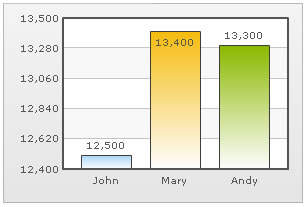You can see above that FusionCharts XT is now showing full numbers on the chart. It has also added commas to the numbers at the required places. If you do not need the commas too, set formatNumber=0. But, setting formatNumber=0 will not format any decimal places too (even if explicitly specified in your data).

Shown below is an example with <chart ... formatNumber='0' formatNumberScale='0' ..> :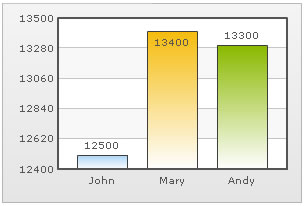You can see that the commas have been removed from numbers.

Again, for Dual y-axis charts, you will have to set it explicitly for secondary axis using the XML/JSON below. While the primary axis, by default, scales numbers to K,M, the secondary axis does not. For the secondary axis to scale to K,M, you will have to explicitly define number scaling for the axis.

`<chart ... formatNumber='0' formatNumberScale='0'  sFormatNumber='0'  sFormatNumberScale='0'...>`
``` "chart":{
...    "formatnumber":"0",    "formatnumberscale":"0",    "sformatnumber":"0",    "sformatnumberscale":"0"
...  }```

Here, the prefix s represents secondary axis.

Setting custom thousand and decimal separator character

By default, FusionCharts XT uses . (period) as decimal separator and , (comma) as thousand separator character. However, you can customize this character depending on your requirements.

To do so, use the decimalSeparator and thousandSeparator attribute. For example, let us set our thousands separator as dot and decimal separator as comma. To do so, you will have to use the following data:

`<chart ... decimalSeparator=',' thousandSeparator='.' > `
```"chart":{
...    "decimalseparator":",",    "thousandseparator":"."  }```

Shown below is the output.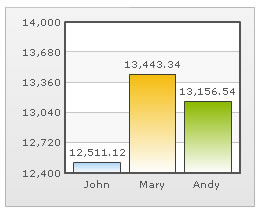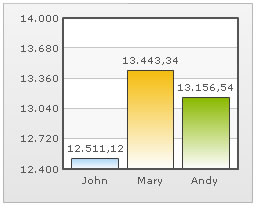Chart with default decimal and thousand separators. FusionCharts XT by default separates thousands using commas and decimals using dots. Chart with swapped decimal and thousands separator character

Configuring thousand separator position

FusionCharts XT automatically decides the position of the thousand separator. By default, the thousand separator is placed after every three digits from right. A chart with default thousand separator position will look as under: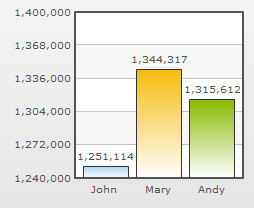Starting FusionCharts XT -Service Release 4, you can customize the position of the thousand separator and place it as required. To place the thousand separator in custom positions you need to use the thousandSeparatorPosition attribute. Shown below is a chart with the thousand separator placed after four digits from right: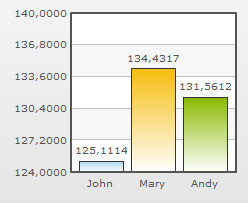In the above chart, the attribute thousandSeparatorPosition is set to '4'. So, the thousand separator (,) is placed after every four digits starting from right.

You can also set multiple values (separated by comma) to this attribute, instead of a single value. Thus, you can specify complex thousand separator positions. For example, specifying multiple values, you can display numbers in Indian currency format, where the first thousand separator (for thousand) is placed after three digits from right and then onwards the thousand separator is placed after every two digits. Shown below is an example chart displaying Indian currency format: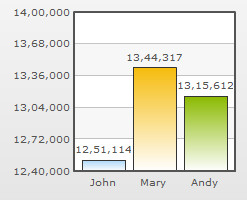In the above chart, we have set thousandSeparatorPosition='2,3'. So, the thousand separator is first placed after 3 digits starting from right and then onwards after every two digits. Given below are some sample combinations of values which can be set to this attribute:

 Value set Description Example thousandSeparatorPosition="0" When this attribute is set to 0, it takes the default value, which is 3, and places the separator after every three digits starting from right. Rule: When zero is specified as the thousand separator position, it assumes the default value, which is 3. 1,344,317,518 thousandSeparatorPosition="2" When this attribute is set to 2, the thousand separator is placed after every two digits starting from right. 13,44,31,75,18 thousandSeparatorPosition="4" When this attribute is set to 4, the thousand separator is placed after every four digits starting from right. 13,4431,7518 thousandSeparatorPosition="1,2" In this case, first the thousand separator is placed after two digits from right. Then onwards the separator will be placed after every digit. 1,3,4,4,3,1,7,5,18 thousandSeparatorPosition="1,2,3" In this scenario, first the separator will be placed after three digits from right, then the separator will be placed after two digits and then onwards it will be placed after every digit. 1,3,4,4,3,17,518 thousandSeparatorPosition="3,1,0" In this scenario, the value 0 will be replaced by the default value 3. So, the thousand separator will be first placed after three digits from right. Then the separator is placed after one digit. Then onwards it will be placed after every three digits. Rule: When zero is specified as the first thousand separator position in a comma separated list of thousand separator positions, it assumes the default value, which is 3. 134,431,7,518 thousandSeparatorPosition="1,0,2" This is a special case where 0 is used as the second value. In this scenario, first the thousand separator is placed after two digits. Next, the value 0 assumes the previous value, which is 2. Hence, the separator will again be placed after two digits and then onwards it will be placed after every digit. Rule: When zero is specified NOT as the first thousand separator position in a comma separated list of thousand separator positions, it assumes the previous value from the list. 1,3,4,4,3,1,75,18 thousandSeparatorPosition="0,1,3" In this scenario, first the thousand separator is placed after three digits. Then it is placed after every digit. Now, the value 0 assumes the previous value, 1 and rest of the separators are placed after every digit. Rule: When zero is specified NOT as the first thousand separator position in a comma separated list of thousand separator positions, it assumes the previous value from the list. 1,3,4,4,3,1,7,518

Note: Any negative value set to this attribute will be converted to a positive value. For example, thousandSeparator="-2" will be converted to thousandSeparator="2".

FusionCharts XT allows you to add a prefix or suffix to all numbers on the chart. You can use the following attributes of <chart> element to attain the same:

• numberPrefix="\$" : Using this attribute, you could add prefix to all the numbers visible on the graph (plotted on primary axis). For example, to represent all dollars figure on the chart, you could specify this attribute to ' \$' to show like \$40000, \$50000.
• numberSuffix="p.a" : Using this attribute, you could add a suffix to all the numbers visible on the graph. For example, to represent all figure quantified as per annum on the chart, you could specify this attribute to ' /a' to show like 40000/a, 50000/a.
• sNumberPrefix="\$" : Using this attribute, you could add prefix to all the numbers visible on the graph (plotted against secondary axis, in case of dual y-axis charts).
• sNumberSuffix="p.a" : Using this attribute, you could add a suffix to all the numbers visible on the graph (plotted against secondary axis, in case of dual y-axis charts).

To know more on how to use currency symbols (£, €, ¥ etc.) refer to Using Currency Symbols page. If you intended to use special punctuation or characters like &, <, >, % etc. refer to Using Special Punctuation page.

Examples: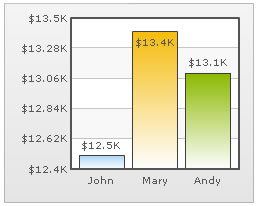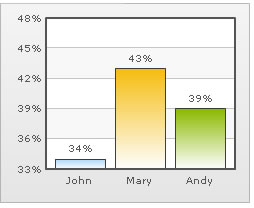Number Prefix set as \$ for the chart Number Suffix Set as %

Decimal formatting for y-axis values when automatic adjustment of div-lines is off

If you have opted to manually set the number of divisional lines on chart, you can also control the decimals of y-axis values separately. For example, consider the following data and chart:

```<chart yAxisMinValue='115' yAxisMaxValue='140' adjustDiv='0' numDivLines='3' >
<set label='John' value='125' />
<set label='Mary' value='134' />
<set label='Andy' value='131' />
</chart>```
`{  "chart":{    "yaxisminvalue":"115",    "yaxismaxvalue":"140",    "adjustdiv":"0",    "numdivlines":"3"  },  "data":[{      "label":"John",      "value":"125"    },    {      "label":"Mary",      "value":"134"    },    {      "label":"Andy",      "value":"131"    }  ]}`

The chart for this XML/JSON looks as under: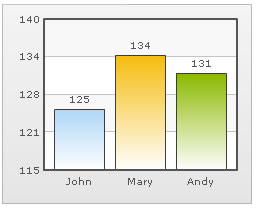In this chart, we have manually fixed the chart lower limit, upper limit and number of divisional lines. We have also asked FusionCharts XT not to automatically adjust divisional lines. Now, if you see the chart, you will find that FusionCharts XT has truncated decimals from y-axis values, as it could not find any other decimal values on the chart.

You can, however, opt to just show decimals on y-axis values in this case (when adjustDiv is false) using forceYAxisValueDecimals='1' and yAxisValueDecimals='number' attribute.

Example:

`<chart yAxisMinValue='115' yAxisMaxValue='140' adjustDiv='0' numDivLines='3' forceYAxisValueDecimals='1' yAxisValueDecimals='2' decimals='0' > `
` "chart":{    "yaxisminvalue":"115",    "yaxismaxvalue":"140",    "adjustdiv":"0",    "numdivlines":"3",    "forceyaxisvaluedecimals":"1",    "yaxisvaluedecimals":"2",    "decimals":"0"  }`

When you now see the chart, you will get 2 decimal places on y-axis values.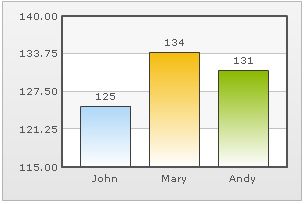For charts with dual y-axis, the XML/JSON will be:

`<chart ...   forceSYAxisValueDecimals='1' SYAxisValueDecimals='2' ...> `
```"chart":{
...    "forcesyaxisvaluedecimals":"1",    "syaxisvaluedecimals":"2"
...  }```

Next, we will see how to utilize the new number scale formatting properties provided in FusionCharts XT.